## Geometric view of Hölder's inequality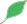This article is a stub.This means that it cannot be considered to contain or lead to any mathematically interesting information.

### Quick description

To understand the standard Hölder inequality better it is helpful to look at a more general version and use a geometric picture of convex bodies.

### Example 1

Say, we want to bound the integral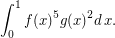Our current knowledge allows us to estimate the integrals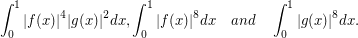How can we see quickly, whether this information is sufficient or not? Secondly, how are we going to choose the coefficients in Hoelder's inequality?

This type of problem is common in analytic number theory, for example.

### General discussion

Hölder's inequality states that on any measure space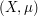, and any measurable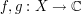, one has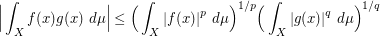whenever we have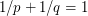.

The key insight here is to view this as an statement about interpolation to get information at the point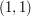from information at the points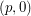and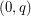. The conditionensures that all three points lie on a line.

Once we have this realisation, it is easy to generalise this to the case of the example above. To obtain information at the point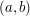, as in the integral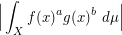it is sufficient to have bounds at three points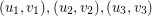such thatlies in the convex hull of the three points.

The coefficients in the estimate are then given by the coefficients of the convex linear combination ofinside the triangle.

The simplicity of the geometric view allows us to handle more complex integrals integrals like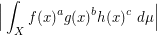with the same ease.

By drawing the corresponding points in the plane, it is easy to judge in advance which of the integrals contributes most to the estimate later, thus providing a tool to estimate the quality of the estimate.